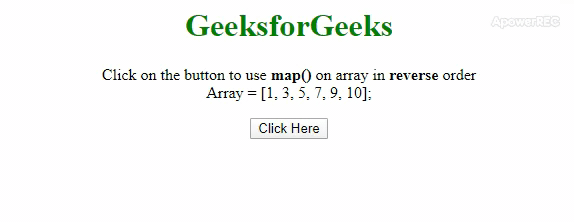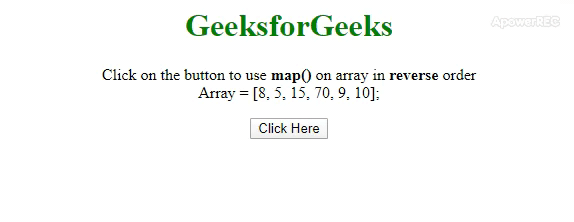# How to use map() on an array in reverse order with JavaScript ?

Given a JavaScript array and the task is to apply the map() method but on the reverse of the array efficiently. Here are a few approaches discussed. If you don’t want to change the original array then you can create a shallow copy of the array after that you can perform the task.

Approach 1: The idea is to use .reverse() method just after applying .slice() method. Then use the .map() method on the reversed array to perform the task.

• Example: This example implements the above approach.

 ` ` `<``html``> ` ` `  `<``head``> ` `    ``<``title``> ` `        ``How to use map() on an array in ` `        ``reverse order with JavaScript ? ` `    `` ` ` `  `    ``<``style``> ` `        ``body { ` `            ``text-align: center; ` `        ``} ` `        ``h1 { ` `            ``color: green; ` `        ``} ` `        ``#geeks { ` `            ``font-weight: bold; ` `        ``} ` `    `` ` ` ` ` `  `<``body``> ` `    ``<``h1``>GeeksforGeeks ` `     `  `    ``<``p``> ` `        ``Click on the button to use ` `        ``<``b``>map() on array in  ` `        ``<``b``>reverse order<``br``> ` `        ``Array = [1, 3, 5, 7, 9, 10]; ` `    `` ` `     `  `    ``<``button` `onclick``=``"gfg_Run()"``> ` `        ``Click Here ` `    `` ` `     `  `    ``<``p` `id``=``"geeks"``> ` `     `  `    ``<``script``> ` `        ``var el_down = document.getElementById("geeks"); ` `        ``var arr = [1, 3, 5, 7, 9, 10]; ` ` `  `        ``/* Main function */ ` `        ``function gfg_Run() { ` `            ``newArr = arr.slice(0).reverse().map( ` `                ``function(val, index) { ` `                    ``return val * 2; ` `                ``} ` `            ``); ` `             `  `            ``el_down.innerHTML = "New Array = [" ` `                                ``+ newArr + "]"; ` `        ``} ` `    `` ` ` ` ` `  ` `

• Output:Approach 2: In this approach we will use the .map() method and call a function inside this method with 2 arguments (value, index). Now we need to access the value, we will access it from reverse side (eg.. arr[arr.length – 1 – index]), this is immutable operation (It doesn’t change the original array).

• Example: This example implements the above approach.

 ` ` `<``html``> ` ` `  `<``head``> ` `    ``<``title``> ` `        ``How to use map() on an array in ` `        ``reverse order with JavaScript ? ` `    `` ` `     `  `    ``<``style``> ` `        ``body { ` `            ``text-align: center; ` `        ``} ` `        ``h1 { ` `            ``color: green; ` `        ``} ` `        ``#geeks { ` `            ``font-weight: bold; ` `        ``} ` `    `` ` ` ` ` `  `<``body``> ` `    ``<``h1``>GeeksforGeeks ` `     `  `    ``<``p``> ` `        ``Click on the button to use  ` `        ``<``b``>map() on array in  ` `        ``<``b``>reverse order<``br``> ` `        ``Array = [8, 5, 15, 70, 9, 10]; ` `    `` ` `     `  `    ``<``button` `onclick``=``"gfg_Run()"``> ` `        ``Click Here ` `    `` ` `     `  `    ``<``p` `id``=``"geeks"``> ` `     `  `    ``<``script``> ` `        ``var el_down = document.getElementById("geeks"); ` `        ``var arr = [8, 5, 15, 70, 9, 10]; ` `         `  `        ``/* Main function */ ` `        ``function gfg_Run() { ` `            ``newArr = arr.map((val, index, array) =>  ` `                    ``1/2*arr[arr.length - 1 - index]); ` `             `  `            ``el_down.innerHTML = "New Array = [" ` `                                ``+ newArr + "]"; ` `        ``} ` `    `` ` ` ` ` `  ` `

• Output:•My Personal Notes arrow_drop_upCheck out this Author's contributed articles.

If you like GeeksforGeeks and would like to contribute, you can also write an article using contribute.geeksforgeeks.org or mail your article to contribute@geeksforgeeks.org. See your article appearing on the GeeksforGeeks main page and help other Geeks.

Please Improve this article if you find anything incorrect by clicking on the "Improve Article" button below.